# NCERT Solutions class-11 Maths Exercise 7.1## myCBSEguide App

CBSE, NCERT, JEE Main, NEET-UG, NDA, Exam Papers, Question Bank, NCERT Solutions, Exemplars, Revision Notes, Free Videos, MCQ Tests & more.

Exercise 7.1

1. How many 3-digit numbers can be formed from the digits 1, 2, 3, 4 and 5 assuming that:

(i) repetition of the digits is allowed

(ii) repetition of the digits is not allowed.

Ans. (i) The unit place can be filled by any one of the digits 1, 2, 3, 4 and 5. Therefore the unit place

can be filled in 5 ways. Similarly, the tens place and hundreds place can be filled in 5 ways each because repetition of digits are allowed.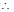Total number of three digit numbers =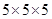= 125

(ii) The unit place can be filled by any one of the digits 1, 2, 3, 4 and 5. Therefore the unit place can be filled in 5 ways. Hence four digits left. So the tens place can be filled in 4 ways. The hundreds place can be filled in 3 ways by the remaining three 3 digits because repetition of digits are not allowed.Total number of three digit numbers =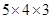= 60

2. How many 3-digit even numbers can be formed from the digits 1, 2, 3, 4, 5, 6 if the digits can be repeated?

Ans. The unit place can be filled by any one of the digits 2, 4, 6. Therefore the unit place can be filled in 3 ways. Now the tens and hundreds places can be filled by any one of the digits 1, 2, 3, 4, 5, 6. Therefore, tens and hundreds places can be filled in 6 ways.Total number of three digit even numbers =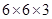= 108

3. How many four-letter codes can be formed using the first 10 letters of the English alphabet, if no letter can be repeated?

Ans. The unit place can be filled by any one of the 10 letters of the English alphabets. So the unit place can be filled in 10 ways. The tens place can be filled in 9 ways by remaining 9 letters of the English alphabets. The hundred place can be filled in 8 ways and the thousand place can be filled in 7 ways.Total number of 4-letter code numbers =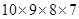= 5040

4. How many 5-digit telephone numbers can be constructed using the digits 0 to 9 if each number starts with 67 and no digit appears more than once?

Ans. The unit place can be filled by any one of the digits 0, 1, 2, , 4, 5, 8, 9. So the unit place can be filled in 8 ways. The tens place can be filled in 7 ways by remaining 7 digits. The hundred place can be filled in 6 ways.Total number of 5-digit telephone numbers =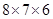= 336

5. A coin is tossed 3 times and the outcomes are recorded. How many possible outcomes are there?

Ans. The coin is tossed first time and the number of outcomes is 2. Now the coin is tossed twice and the number of outcomes is 2 again. Also the coin is tossed thrice and the number of outcomes is 2 again.Total number of outcomes in tossing a coin 3 times =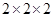= 8

6. Given 5 flags of different colours, how many different signals can be generated if each signal requires the use of 2 flags, one below the other?

Ans. The upper place of the flag can be filled in 5 ways by using the 5 flags of different colours. Now the lower place of the flag can be filled in 4 ways by using the remaining 4 flags of different colours.Total number of signals =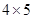= 20### 3 thoughts on “NCERT Solutions class-11 Maths Exercise 7.1”

1. 7.2 question

2. Up board

3. i impres with this answer